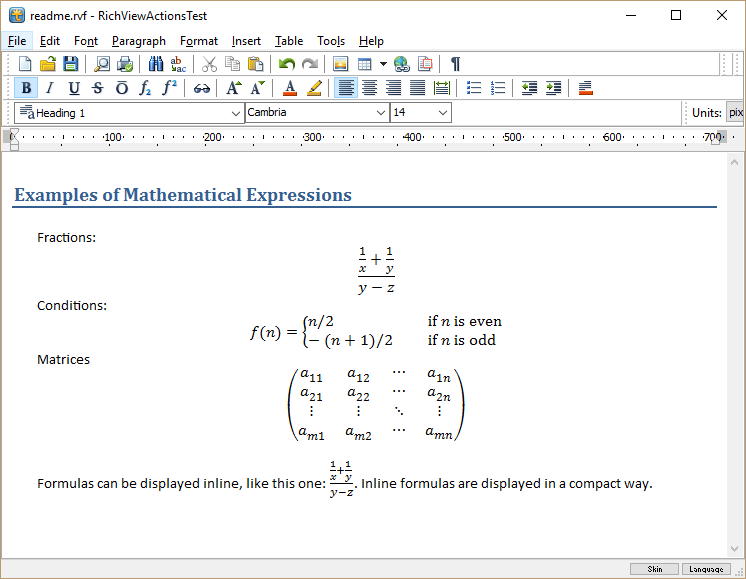Mathematical expressions in TRichView

News about TRichView, ScaleRichView, ReportWorkshop, RVMedia
Sergey Tkachenko
Posts: 14634
Joined: Sat Aug 27, 2005 10:28 am
Contact:

Mathematical expressions in TRichView

Starting from TRichView v16.14, a new item type can be added in documents: mathematical expressions (i.e. equations, formulas, etc.)
This item's type is TRVMathItemInfo.
Expressions are defined in a LaTeX-like language.The current version includes the item itself, but does not include a dialog for editing equations. It will be included in the next update.

The item uses units of Adit Math Engine. Currently, they are distributed exclusively with TRichView.
They are covered by the open source license MPL 2.0 with the following addition restrictions:
Adit Math Engine cannot be used in any E-learning/Assessment/Testing/Math software (Freeware or Shareware) or outside TRichView engine without our [Adit Software] written permission.
You can contact Adit Software if you want to use Adit Math Engine under a different license or to request an exception from the restrictions above.
The main reason of these restrictions is not allowing using in software that can compete with their own product.
Currently, Adit Math Engine can be used in RAD Studio XE4 and newer. Contact them if you want to use it in older versions of Delphi/C++Builder.

Sergey Tkachenko
Posts: 14634
Joined: Sat Aug 27, 2005 10:28 am
Contact:

Re: Mathematical expressions in TRichView

Properties

The main properties of this item are: font name, font size (defined in half-points), text color. You can set default values for all formulas in the document, and override them in specific formulas.

You can also define fill color, border color and width.

You can also specify the inline style for the formula (Boolean property). The inline style is more compact and is recommended for using for formulas inserted in text lines.

You can use only mathematical fonts. By default, the item uses 'Cambria Math'. You can use other mathematical fonts as well (such as 'Asana Math', 'Latin Modern Math', 'XITS Math', see the help file for download locations).
If the specified font is not installed on the computer, the item uses 'Cambria Math'. If 'Cambria Math' is not installed, it uses the first found available mathematical font. If no mathematical font is found, the item is displayed in an erroneous state (a crossed rectangle).
Note: 'Cambria Math' is a default font for equations in Microsoft Word.

Export
This version of the item is exported to HTML, RTF and DocX as a raster image (if possible, as PNG). Probably, more options will be available in future.

Sergey Tkachenko
Posts: 14634
Joined: Sat Aug 27, 2005 10:28 am
Contact:

Re: Mathematical expressions in TRichView

Expressions used in the screenshot in this post:

Code: Select all

\frac{\frac{1}{x}+\frac{1}{y}}{y-z}

f(n) = \case{ n/2 & \quad \text{if } n \text{ is even} \\ -(n+1)/2  & \quad \text{if } n \text{ is odd}}

\matrix{ a_{11} & a_{12} & \cdots & a_{1n} \\ a_{21} & a_{22} & \cdots & a_{2n} \\ \vdots & \vdots & \ddots & \vdots \\ a_{m1} & a_{m2} & \cdots & a_{mn} }
More about LaTeX formulas: https://en.wikibooks.org/wiki/LaTeX/Mathematics
Note that the item's language has differences in defining matrices and cases.

We plan to add a dialog for visual editing of these strings in RichViewActions soon.

DmitriPopov
Posts: 29
Joined: Tue Dec 15, 2009 10:01 am

Re: Mathematical expressions in TRichView

Good news!
But what was the reason behind making formulas "LaTeX-like", and not just LaTeX? Users are accustomed to LaTeX, there's no need to define a subgenre. Another problem is that it's impossible to paste LaTeX code from another source, it needs conversion.

Sergey Tkachenko